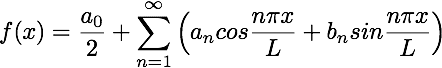# How is the constant pi/L deduced in Fourier series?

• I
• henry wang

#### henry wangHow is pi/L part deduced in (n*pi*x)/L?

Essentially it has to do with the interval that the series is defined for. The length of the interval is 2L while $\pi$ is the normalization (based on the fact that sines and cosines have period $2\pi$).

•henry wang
henry wang said:How is pi/L part deduced in (n*pi*x)/L?

We're requiring that the fundamental frequency is periodic in space, with spatial period ##2L##. So we have for the fundamental frequency: $$\cos \omega x = \cos(\omega(x+2L)) = \cos(\omega x + 2L \omega) \Rightarrow 2L \omega = 2\pi \Rightarrow \omega = \frac{\pi}{L}$$

•henry wang
•henry wang
Thank you guys, I understand now.